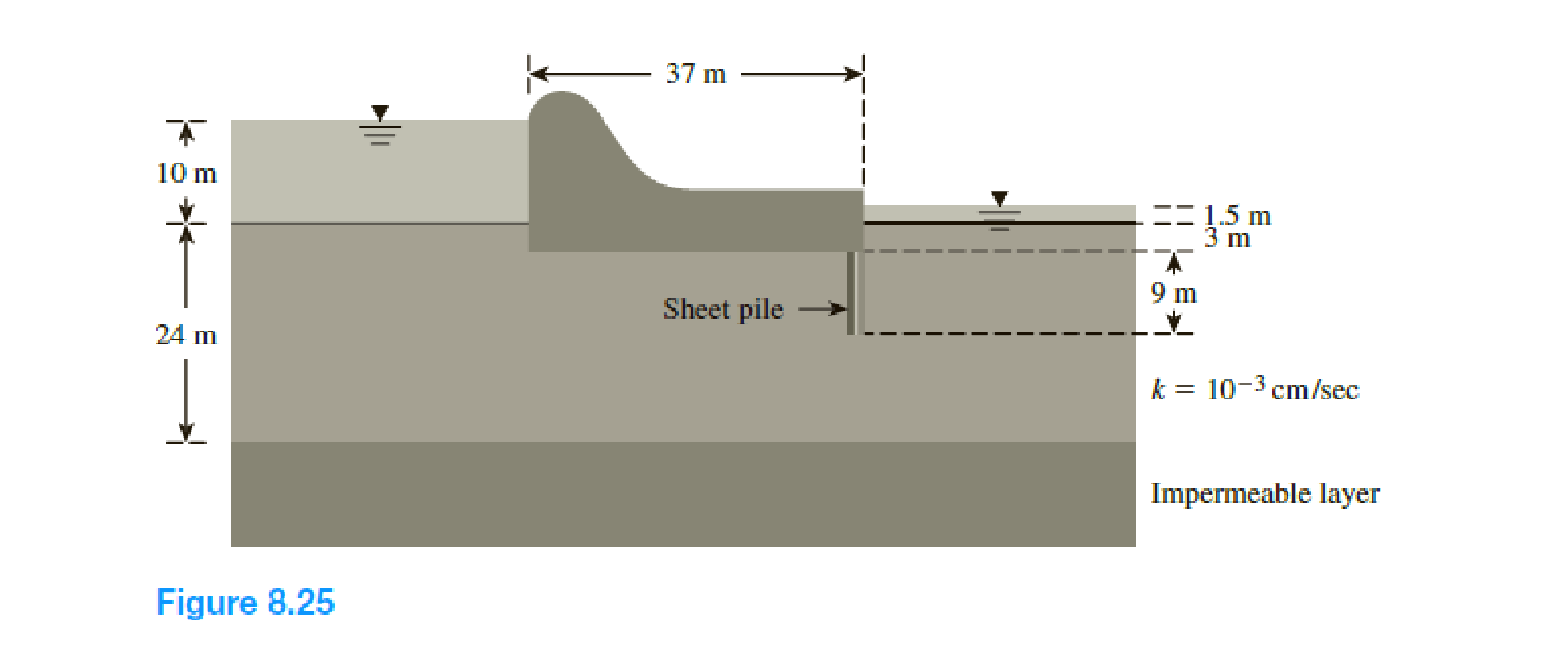Chapter 8, Problem 8.6PPrinciples of Geotechnical Enginee...

9th Edition
Braja M. Das + 1 other
ISBN: 9781305970939

Solutions

Chapter
SectionPrinciples of Geotechnical Enginee...

9th Edition
Braja M. Das + 1 other
ISBN: 9781305970939
Textbook Problem

Draw a flow net for the weir shown in Figure 8.25. Calculate the rate of seepage under the weir.To determine

Draw the flow net for the weir and calculate the seepage under the weir.

Explanation

Given information:

The hydraulic conductivity of the permeable soil layer k is 103cm/sec.

The head difference between the upstream and downstream H1 is 10 m.

The height of the water level H2 is 1.5 m.

The depth of permeable layer up to the tip of the hydraulic structure D is 11 m.

The depth of permeable layer D1 is 24 m.

Calculation:

Draw the free body diagram of the flow net for the given values as in Figure 1.

Determine the head difference between the upstream and downstream using the relation.

H=H1H2

Substitute 10 m for H1 and 1.5 m for H2.

H=101.5=8.5m

Determine the seepage loss per meter length of the sheet pile using the relation.

q=kHNfNd

Here, Nf is the number of flow lines and Nd is the number of potential drops

Still sussing out bartleby?

Check out a sample textbook solution.

See a sample solution

The Solution to Your Study Problems

Bartleby provides explanations to thousands of textbook problems written by our experts, many with advanced degrees!

Get Started

What basic factors affect the required time to recharge the battery pack in a BEV?

Automotive Technology: A Systems Approach (MindTap Course List)

What are the two spindle types used for surface grinders?

Precision Machining Technology (MindTap Course List)

If such an e-mail came in, what would be the best action to take?

Principles of Information Security (MindTap Course List)

What are the basic characteristics of a NoSQL database?

Database Systems: Design, Implementation, & Management

Define a class, subclass, and superclass, and provide three examples of each.

Systems Analysis and Design (Shelly Cashman Series) (MindTap Course List)

Which type of light is the most likely to cause burns? Why?

Welding: Principles and Applications (MindTap Course List)